Latest Teaching jobs   »   UGC NET Commerce Notes   »   Theory of Cost

# Theory of Cost: Meaning & Types

Cost theories include the study of the behaviour of cost with respect to various production criteria like the scale of operations, prices of the factors of production, size of output, etc. It is all about the financial aspects of production. In this article, we are going to discuss in detail the topic of cost, its types and different cost theories.

## Theory of Cost Overview

Determining the price of a product or service is not easy. Several other factors also affect this. Cost Description Theory states that a firm’s costs largely determine its inputs and expenditures. Concepts such as cost theory, cost, full discount and average cost, long-term cost and economies of scale play an important role in modern economic studies.

## Meaning of Cost

Cost can be defined as the cost of all the sacrifices (e.g. production and service) made to achieve the goal. Product Value is paramount in business decisions. Production costs make up the bottom line of the product.

It helps managers make decisions such as what price to bid, whether to order ideas, whether to launch products or to add products to existing ones.

## Types of cost

Cost can be classified into different categories. These are as follows

• Accounting cost/ explicit cost: This cost of production consists of employee salaries, raw material costs, rent expenses fuel costs, and all the payments made to the suppliers from the accounting costs. This is also called explicit cost.
• Economic cost/ implicit cost: Implicit cost refers to the amount that could have been earned but actually not paid to an entrepreneur. This includes monetary rewards for all estates owned by the businessman form the economic costs.
• Outlay Costs: This is the actual expenditure spent on wages, rent, raw materials and more.
• Opportunity Costs: This cost belongs to the missed opportunity where the money could have been spent. They are not recorded in the account books but reflect the cost of sacrificed or rejected policies.
• Direct / Traceable Costs: These costs are easily identified as expenditures such as manufacturing costs. Such costs relate directly to the specific operations or goods.
• Indirect / Non-Traceable Costs: These costs are not directly related to or associated with the work or service. Costs such as electricity or water leakage are some examples of indirect costs that vary with the volume of production. Most of these are production-related.
• Fixed Costs: Such costs do not change with a change in output and are fixed expenditures of the company. For example, taxes, rent, and interests are all fixed costs as they do not vary within a constant capacity. No company cannot avoid these costs.
• Variable Costs: These costs vary with the change in output and are known as variable costs. For example, raw material costs and the salaries of the employee, all fall under variable costs. These directly depend on the fixed amount of resources.
• Direct / Traceable Costs: These costs are easily identified as expenditures such as manufacturing costs. Such costs cater to specific operations or goods.
• Indirect / Non-Traceable Costs: These costs are not directly related to or associated with the work or service. Costs such as electricity or water leakage are some examples as they vary with the volume of production. They often have a relationship with production.

## Theories of cost

There are two theories of cost. One is the traditional theory and another is the modern theory of cost. Below we will discuss these two in detail

In traditional theory, costs are generalized in two parts on the basis of time period i.e. costs in the short run and costs in the long run period.

Cost in the short run can be divided into three categories

• Total cost
• Average cost
• Marginal cost

#### Total Cost

According to Dooley, “Total cost of production is the aggregate of all expenditure incurred in producing a given volume of output.”

• Fixed Costs or Supplementary Costs

The cost that stays constant at any level of output is known as the fixed cost. These costs must have to be paid whether there is production of output or not.

• Variable Costs or Prime Costs

Variable costs refer to those costs which alter with the change in the volume of production output. These are unavoidable or contractual costs. Marshall termed these costs as “Prime Costs”, “Direct Costs” or “Special Costs”.

Relation between Total, Fixed and Variable Costs:

In order to determine the total costs of a firm, we accumulate aggregate fixed as well as variable costs at distinct levels of outputs i.e.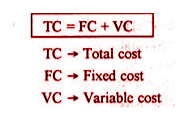#### Average Cost

According to Dooley, “The average cost of production is the total cost per unit of output produced.” In other words, the average cost of production is the aggregate cost of production divided by the total number of units produced.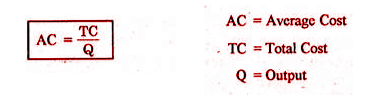In the short-run average cost curves are of U-shape. It represents, initially it falls and after reaching the minimum level it begins rising upwards.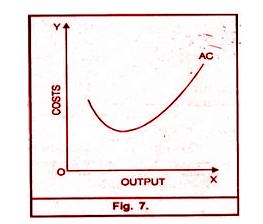#### Marginal Cost

Marginal cost is an addition to the total cost caused by producing one more unit of output. For instance, the total cost for the production of 100 units is Rs. 4000. Suppose the production of one more unit costs Rs.50. This addition to the cost is called the marginal cost.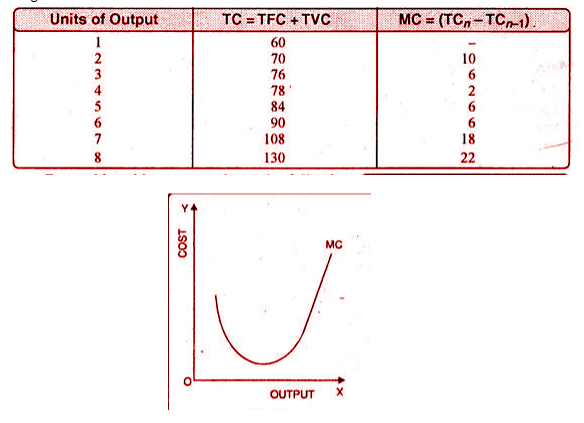### Modern Theories of Cost

Modern theories of cost build upon and extend the classical or traditional theories of cost, incorporating new insights, mathematical modelling, and empirical research. These theories are essential for understanding how firms make production decisions, set prices, and optimize their operations in various market structures.

#### Cost Output Relationship in the Long Run

In order to study the relationship of cost-output in the long run it is important to understand the meaning of the long run.

In the long run, the size of an industry can be expanded as per the requirement in order to meet the increased demand for products. This can be done in the long run since no factor of production in the long run is fixed. We can vary the factors as per the requirement.

#### Features of the long-run cost curve

In the long run, the cost curves have the following features

• The LAC (long-run average cost) curve envelopes the SAC (short-run average cost) curves and is hence called the envelope curve.
• The points of tangency are on the falling part of the SAC curve for points fibbing to the left of the minimum point of LAC.
• The points of tangency occur on the rising part of the SAC curves for the points lying to the right of the minimum point of LAC
• Both LAC and SAC curves are U-shaped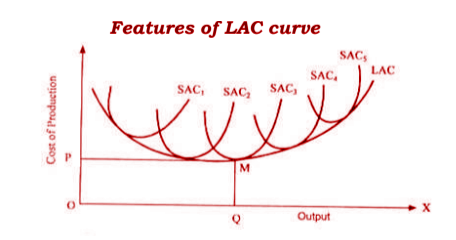## Conclusion

Hence, the theory of cost assists in determining various components and aspects of cost. Understanding cost becomes important because cost is the main element of pricing a product or service. Cost theory helps in effective estimation and understanding of the cost element.

Sharing is caring!

## FAQs

### What is the Theory of Cost?

The Theory of Cost explores the behavior of costs concerning factors like production scale, input prices, and output size. It is a crucial aspect of economics that deals with the financial aspects of production and decision-making.

### What is the Meaning of Cost in Economics?

In economics, cost refers to all the sacrifices, including production and service, made to achieve a goal. It plays a pivotal role in business decisions, affecting pricing, product launches, and resource allocation.

### What are the Types of Costs?

Costs can be categorized into various types, including accounting cost, economic cost, outlay costs, opportunity costs, fixed costs, variable costs, direct costs, and indirect costs.

### What are Fixed Costs and Variable Costs?

Fixed costs remain constant regardless of the level of production, while variable costs change with changes in output. Examples of fixed costs include rent and taxes, while raw materials and employee salaries are variable costs.

### How does the Long-Run Cost Curve differ from the Short-Run Cost Curve?

In the long run, the size of an industry can be adjusted to meet increased demand, making all factors of production variable. Long-run cost curves envelop short-run cost curves and have specific features like U-shape and tangency points.

### Why is the Theory of Cost Important?

The Theory of Cost is essential for pricing decisions, resource allocation, and understanding the cost elements of products and services. It helps firms estimate and manage costs effectively.